Updating search results...

# 39 Results

View
Selected filters:
• NC.Math.8.F.4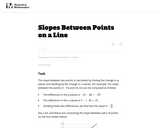Unrestricted Use
CC BY
Rating
0.0 stars

This is a task from the Illustrative Mathematics website that is one part of a complete illustration of the standard to which it is aligned. Each task has at least one solution and some commentary that addresses important asects of the task and its potential use. Here are the first few lines of the commentary for this task: The slope between two points is calculated by finding the change in \$y\$-values and dividing by the change in \$x\$-values. For example, the slope between...

Subject:
Mathematics
Material Type:
Activity/Lab
Provider:
Illustrative Mathematics
Provider Set:
Illustrative Mathematics
Author:
Illustrative Mathematics
08/19/2013Conditional Remix & Share Permitted
CC BY-NC
Rating
0.0 stars

Learning Pathway for 8.F.4Quiz links will need to be added for assessing purposes.

Subject:
Mathematics
Material Type:
Activity/Lab
Author:
ERIN WOLFHOPE
04/06/2020Rating
0.0 stars

The Common Core State Standards (CCSS) videos are designed to support states, schools, and teachers in the implementation of selected math standards. Each video is an audiovisual resource that focuses on one or more specific standards through examples and illustrations geared to enhancing understanding. The intent of each content-focused video is to clarify the meaning of the individual standard rather than to be a guide on how to teach each standard, although the examples can be adapted for instructional use.

Subject:
Mathematics
Material Type:
Lesson
Provider:
The Southeast Comprehensive Center
Author:
The Southeast Comprehensive Center
02/26/2019Conditional Remix & Share Permitted
CC BY-NC-SA
Rating
0.0 stars

This 8th grade Math&nbsp;parent guide&nbsp;explains the content in straightforward terms so they can support their children&rsquo;s learning at home and will&nbsp;encourage&nbsp;caretaker engagement&nbsp;with lessons.

Subject:
Mathematics
Material Type:
Reference Material
Author:
Kelly Rawlston
Letoria Lewis
01/10/2022Conditional Remix & Share Permitted
CC BY-NC-SA
Rating
0.0 stars

Our Teacher Guides are meant to support the use of our online course and unit content. Please use these to accompany the use of our content and for ideas to support struggling learners, those needing extension and for additional resources.

Subject:
Mathematics
Material Type:
Curriculum
Teaching/Learning Strategy
Author:
Kelly Rawlston
Letoria Lewis
05/16/2022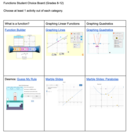Unrestricted Use
Public Domain
Rating
0.0 stars

Students will complete a Functions Choice Board they will have two options from each section. What is a function, graphing linear functions and Graphing Quadratic.

Subject:
Mathematics
Material Type:
Activity/Lab
Author:
Tyler Bridgers
02/24/2022Educational Use
Rating
0.0 stars

In this interactive, web-based game, students must determine the slope-intercept form of a line to destroy cockroaches marching across the coordinate grid.

Subject:
Mathematics
Material Type:
Activity/Lab
Provider:
CPALMS
Author:
Hans Software/Hotmath.com
02/26/2019Conditional Remix & Share Permitted
CC BY-NC
Rating
0.0 stars

In CK-12 Middle School Math Concepts Grade 8, the learning content is divided into concepts. Each concept is complete and whole providing focused learning on an indicated objective. Theme-based concepts provide students with experiences that integrate the content of each concept. Students are given opportunities to practice the skills of each concept through real-world situations, examples, guided practice and explore more practice. There are also video links provided to give students an audio/visual way of connecting with the content.

Subject:
Mathematics
Material Type:
Full Course
Lesson Plan
Textbook
Unit of Study
Provider:
CK-12 Foundation
Provider Set:
CK-12 FlexBook
12/06/2018Conditional Remix & Share Permitted
CC BY-NC-SA
Rating
0.0 stars

In this lesson, students determine a linear function given a verbal description of a linear relationship between two quantities. Students interpret linear functions based on the context of a problem. Students graph linear functions by constructing a table of values, plotting points, and drawing the line.

Subject:
Mathematics
Material Type:
Lesson Plan
Provider:
EngageNY
02/21/2017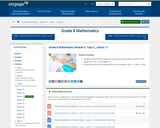Conditional Remix & Share Permitted
CC BY-NC-SA
Rating
0.0 stars

In this lesson, students show that the slope of a line joining any two distinct points of the graph of y = mx + b has slope, m. Students transform the standard form of an equation into y = -(a/b)x + (c/b).

Subject:
Mathematics
Material Type:
Lesson Plan
Provider:
EngageNY
02/20/2017Unrestricted Use
CC BY
Rating
0.0 stars

Function end of unit review function board.&nbsp;

Subject:
Mathematics
Material Type:
Activity/Lab
Author:
Tyler Bridgers
11/16/2021Rating
0.0 stars

This packet contains a curriculum-embedded Common Core?aligned task and instructional supports. The task is embedded in a 2?3 week unit on proportional relationships, linear equations, and slopes. In this unit, students will build on previous work with proportional relationships and unit rates. Students will deepen their understanding of functions and linear equations. In this series of tasks, students will graph proportional relationships and interpret the unit rate as the slope of the line. In addition to deriving and interpreting the equation y = mx + b, students will construct a function to model a linear relationship between two quantities. Students will also explore similar triangles and use them to explain why the slope is constant between any two points on a given line.

Subject:
Mathematics
Material Type:
Activity/Lab
Assessment
Unit of Study
Provider:
The New York City Department of Education
Author:
The New York City Department of Education
02/26/2019Rating
0.0 stars

Students will extend what they already know about unit rates and proportional relationships to linear equations and their graphs. Students understand the connections between proportional relationships, lines, and linear equations in this module.

Subject:
Mathematics
Material Type:
Unit of Study
Provider:
EngageNY
Author:
EngageNY
02/26/2019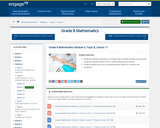Conditional Remix & Share Permitted
CC BY-NC-SA
Rating
0.0 stars

In this lesson, students know the definition of constant rate in varied contexts as expressed using two variables where one is t representing a time interval. Students graph points on a coordinate plane related to constant rate problems.

Subject:
Mathematics
Material Type:
Lesson Plan
Provider:
EngageNY
02/17/2017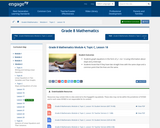Conditional Remix & Share Permitted
CC BY-NC-SA
Rating
0.0 stars

In this lesson, students prove that any point on the graph of y = mx + b is on a line l and that any point on a line l is a point on the graph of y = mx + b. Students graph linear equations on the coordinate plane.

Subject:
Mathematics
Material Type:
Lesson Plan
Provider:
EngageNY
02/20/2017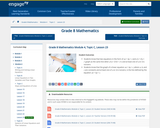Conditional Remix & Share Permitted
CC BY-NC-SA
Rating
0.0 stars

In this lesson, students know that two equations in the form of ax + by = c and a'x + b'y = c' graph as the same line when a'/a = b'/b = c'/c and at least one of a or b is nonzero. Students know that the graph of a linear equation ax + by = c, where a, b, and c are constants and at least one of a or b is nonzero, is the line defined by the equation ax + by = c.

Subject:
Mathematics
Material Type:
Lesson Plan
Provider:
EngageNY
02/20/2017Conditional Remix & Share Permitted
CC BY-NC-SA
Rating
0.0 stars

In this lesson, students graph linear equations in standard form, ax + by = c (a or b = 0), that produce a horizontal or a vertical line.

Subject:
Mathematics
Material Type:
Lesson Plan
Provider:
EngageNY
02/17/2017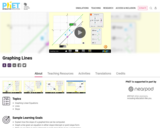Unrestricted Use
CC BY
Rating
0.0 stars

Sample Learning Goals
Explain how the slope of a graphed line can be computed.
Graph a line given an equation in either slope-intercept or point-slope form.
Write an equation in slope-intercept or point-slope form given a graphed line.
Predict how changing variables in a linear equation will affect the graphed line.

Subject:
Mathematics
Material Type:
Activity/Lab
Author:
PhET Interactive Simulations
10/05/2021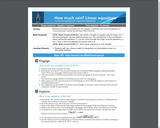Conditional Remix & Share Permitted
CC BY-NC-SA
Rating
0.0 stars

Measure the distance between two rain gauges to estimate how much precipitation an intervening town receives by deriving a linear function.

Subject:
Mathematics
Material Type:
Activity/Lab
Author:
ESRI
04/11/2020Rating
0.0 stars

Students will participate in this discovery activity intended for them to uncover the role each variable plays in the graph of a line in the form y = mx + b. Students will actually demonstrate lines in slope intercept form on a life size graph. They will compare different graphs to see what effect adding negative signs and coefficients to the variables have on the graph. They will also analysis what happens to the graph when a constant is added or subtracted from the variable.

Subject:
Mathematics
Material Type:
Lesson Plan
Provider:
Alabama Learning Exchange
Author:
Joquitta Posey